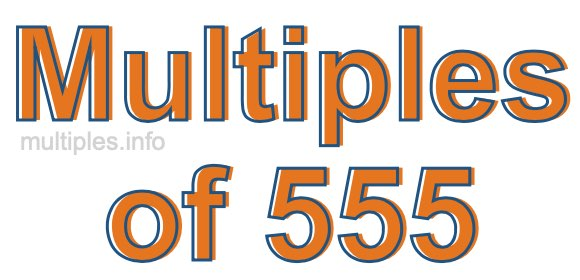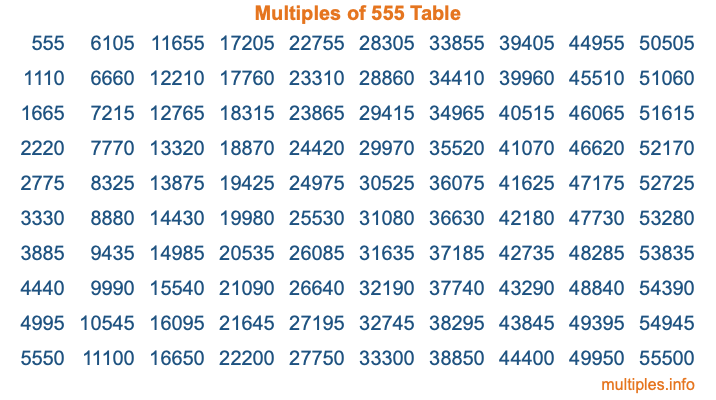Multiples of 555Welcome to the Multiples of 555 page. Here we will first teach you everything you will ever need to know about the multiples of 555, and then give you a study guide summary of everything we taught you to make sure you remember it all. Use this page to look up facts and learn information about the multiples of 555. This page will make you a multiples of five hundred fifty-five expert!

Definition of Multiples of 555
Multiples of 555 are all the numbers that when divided by 555 equal an integer. Each of the multiples of 555 are called a multiple. A multiple of 555 is created by multiplying 555 by an integer.

Therefore, to create a list of multiples of 555, you start with 1 multiplied by 555, then 2 multiplied by 555, then 3 multiplied by 555, and so on for as long as you want. Thus, the list of the first five multiples of 555 is 555, 1110, 1665, 2220, and 2775. To see a larger list of multiples of 555, see the printable image of Multiples of 555 further down on this page. We also have a category where you can choose any nth multiple of 555.

Multiples of 555 Checker
The Multiples of 555 Checker below checks to see if any number of your choice is a multiple of 555. In other words, it checks to see if there is any number (integer) that when multiplied by 555 will equal your number. To do that, we divide your number by 555. If the the quotient is an integer, then your number is a multiple of 555.

Is  a multiple of 555?

Least Common Multiple of 555 and ...
A Least Common Multiple (LCM) is the lowest multiple that two or more numbers have in common. This is also called the smallest common multiple or lowest common multiple and is useful to know when you are adding our subtracting fractions. Enter one or more numbers below (555 is already entered) to find the LCM.

Check out our LCM Calculator if you need more details about the Least Common Multiple or if you need the LCM for different numbers for adding and subtraction fractions.

nth Multiple of 555
As we stated above, 555 is the first multiple of 555, 1110 is the second multiple of 555, 1665 is the third multiple of 555, and so on. Enter a number below to find the nth multiple of 555.

th multiple of 555

Multiples of 555 vs Factors of 555
555 is a multiple of 555 and a factor of 555, but that is where the similarities end. All postive multiples of 555 are 555 or greater than 555. All positive factors of 555 are 555 or less than 555.

Below is the beginning list of multiples of 555 and the factors of 555 so you can compare:

Multiples of 555: 555, 1110, 1665, 2220, 2775, etc.

Factors of 555: 1, 3, 5, 15, 37, 111, 185, 555

As you can see, the multiples of 555 are all the numbers that you can divide by 555 to get a whole number. The factors of 555, on the other hand, are all the whole numbers that you can multiply by another whole number to get 555.

It's also interesting to note that if a number (x) is a factor of 555, then 555 will also be a multiple of that number (x).

Multiples of 555 vs Divisors of 555
The divisors of 555 are all the integers that 555 can be divided by evenly. Below is a list of the divisors of 555.

Divisors of 555: 1, 3, 5, 15, 37, 111, 185, 555

The interesting thing to note here is that if you take any multiple of 555 and divide it by a divisor of 555, you will see that the quotient is an integer.

Multiples of 555 Table
Below is an image of the first 100 multiples of 555 in a table. The table is in chronological order, column by column. The first column has the first ten multiples of 555, the second column has the next ten multiples of 555, and so on.The Multiples of 555 Table is also referred to as the 555 Times Table or Times Table of 555. You are welcome to print out our table for your studies.

Negative Multiples of 555
Although not often discussed or needed in math, it is worth mentioning that you can make a list of negative multiples of 555 by multiplying 555 by -1, then by -2, then by -3, and so on, to get the following list of negative multiples of 555:

-555, -1110, -1665, -2220, -2775, etc.

Multiples of 555 Summary
Below is a summary of important Multiples of 555 facts that we have discussed on this page. To retain the knowledge on this page, we recommend that you read through the summary and explain to yourself or a study partner why they hold true.

There are an infinite number of multiples of 555.

A multiple of 555 divided by 555 will equal a whole number.

555 divided by a factor of 555 equals a divisor of 555.

The nth multiple of 555 is n times 555.

The largest factor of 555 is equal to the first positive multiple of 555.

555 is a multiple of every factor of 555.

555 is a multiple of 555.

A multiple of 555 divided by a divisor of 555 equals an integer.

555 divided by a divisor of 555 equals a factor of 555.

Any integer times 555 will equal a multiple of 555.

Multiples of a Number
Here you can get the multiples of another number, all with the same attention to detail as we did for multiples of 555 on this page.

Multiples of
Multiples of 556
Did you find our page about multiples of five hundred fifty-five educational? Do you want more knowledge? Check out the multiples of the next number on our list!